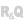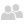Home |Sign in | EnglishHelpWLanguageWLanguage functionsStandard functionsNumeric values management functionsMatrix functionsThe matricesOverviewDefinitionHow to handle matrices?Related examplesSee alsoFunctions for managing matricesMatAddMatCopyMatCreateMatDeleteMatDeterminantMatErrorMatExistMatFillMatFloatAddMatFloatMultiplyMatInvertMatMultiplyMatNbColumnMatNbLineMatReadMatReadColumnMatReadLineMatStackMatTransposeMatWriteThe matrices
• Overview
• Definition
• How to handle matrices?
WINDEVWEBDEVWINDEV MobileOthersThe matrices
Overview
WINDEV, WINDEV Mobile and WEBDEV propose several functions used to handle matrices.
The matrices are mathematical tools used to simplify and solve several problems. The calculations on matrices are used in many fields: economy, physics, ...
In these fields, the problems can often be formulated as a set of equations. The result of this set of equations can be calculated via the matrices.
Definition
A matrix is an organized set of "n p" numbers, displayed in an array of n rows and p columns.
The numbers included in the matrix are the matrix elements. Each element is identified by its row and by its column.
A value is associated with each element.In this matrix, n=2 rows and p=4 columns. The value of the element (row 2 , column 3) is 7
The dimension (or size) of the matrix corresponds to the number of rows and to the number of columns found in the matrix.
WINDEV, WINDEV Mobile et WEBDEV allow you to manage the size of your matrices dynamically. Indeed, a matrix is empty when it is created: it contains 0 row and 0 column. The dimension of this matrix is 0 x 0.
When an element is initialized in row n and column p, the matrix size becomes n x p.
For example, when an element is initialized in row 6 and column 12, the matrix size becomes 6 x 12.
How to handle matrices?
WINDEV, WINDEV Mobile and WEBDEV propose about 20 WLanguage functions for managing matrices. These functions are used to:
• handle the matrices: creation, copy, inversion, compression, deletion.
• initialize the elements of matrices.
• read the value of elements found in the matrices.
• perform various calculations on the matrices: addition, multiplication, transpose, determinant, ...
• get information about the matrices: number of rows, number of columns, ...
Related Examples:Unit examples (WINDEV): The Stat functions [ + ] Using the main functions for statistical calculations (using matrices):- Calculate a sum of values- Calculate a mean of values- Find the minimum value in a set of values- Find the maximum value in a set of valuesUnit examples (WEBDEV): The Stat functions [ + ] This example presents the main functions for statistical calculations (using matrices) of WEBDEV and it allows you to:- Calculate a sum of values- Calculate an average of values- Find the minimum value in a series of values- Find the maximum value in a series of valuesUnit examples (WINDEV Mobile): The Stat functions [ + ] Using the main functions for statistical calculations (using matrices):- Calculate a sum of values- Calculate a mean of values- Find the minimum value in a set of values- Find the maximum value in a set of values